# Math logic

There are 20 children in the group, each two children have a different name. Alena and John are among them. How many ways can we choose 8 children to be among the selected

A) was John
B) was John and Alena
C) at least one was Alena, John
D) maximum one was Alena, John?

Correct result:

a =  50388
b =  18564
c =  75582
d =  196035840

#### Solution: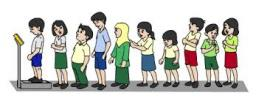We would be pleased if you find an error in the word problem, spelling mistakes, or inaccuracies and send it to us. Thank you!Tips to related online calculators
Would you like to compute count of combinations?

## Next similar math problems:

• Honored students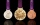Of the 25 students in the class, 10 are honored. How many ways can we choose 5 students from them, if there are to be exactly two honors between them?
• Two groupsThe group of 10 girls should be divided into two groups with at least 4 girls in each group. How many ways can this be done?
• Pairs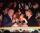At the table sit 10 people, 5 on one side and 5 on the other side. Among them are 3 pairs. Every pair wants to sit opposite each other. How many ways can they sit?
• Candy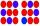How many ways can divide 10 identical candies to 5 children?
• ClassroomOf the 26 pupils in the classroom, 12 boys and 14 girls, four representatives are picked to the odds of being: a) all the girls b) three girls and one boy c) there will be at least two boys
• Toys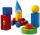3 children pulled 12 different toys from a box. Many ways can be divided toys so that each children had at least one toy?
• Competition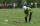15 boys and 10 girls are in the class. On school competition of them is selected 6-member team composed of 4 boys and 2 girls. How many ways can we select students?
• Raffle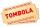There are 200 draws in the raffle, but only 20 of them win. What is the probability of at least 4 winnings for a group of people who have bought 5 tickets together?
• Combinations of sweatersI have 4 sweaters two are white, 1 red and 1 green. How many ways can this done?On the menu are 12 kinds of meal. How many ways can we choose four different meals into the daily menu?
• School parliament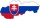There are 18 boys and 14 girls in the class. In how many ways can 3 representatives be elected to the school parliament, if these are to be: a) the boys themselves b) one boy and two girlsThere are 15 boys and 12 girls at the graduation party. Determine how many four couples can be selected.There are 20 boys and 10 girls in the class. How many different dance pairs can we make of them?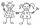From the five girls and four boys teachers have to choose one pair of boy and girl. A) How many such pairs of (M + F)? B) How many pairs where only boys (M + M)? C) How many are all possible pairs?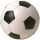How many matches will be played in a football tournament in which there are two groups of 5 teams if one match is played in groups with each other and the group winners play a match for the overall winner of the tournament?How many different ways can three people divide 7 pears and 5 apples?A student is to answer 8 out of 10 questions on the exam. a) find the number n of ways the student can choose 8 out of 10 questions b) find n if the student must answer the first three questions c) How many if he must answer at least 4 of the first 5 ques